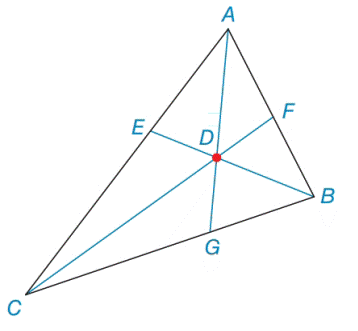Chapter 7.2, Problem 40EElementary Geometry For College St...

7th Edition
Alexander + 2 others
ISBN: 9781337614085

Solutions

Chapter
SectionElementary Geometry For College St...

7th Edition
Alexander + 2 others
ISBN: 9781337614085
Textbook Problem

In Δ A B C , the altitudes are concurrent at point D . If m ∠ E D A = 54 ∘ and m ∠ F D B = 59 ∘ , find m ∠ A C B .Exercises 39, 40

To determine

To find:

the angle mACB in triangle ABC.

Explanation

Definition:

Altitudes of a triangle:

An altitudes is a line which passes through a vertex of a triangle, and meets the opposite side at right angles.

Given:

In ΔABC, the altitudes are concurrent at point D. If mEDA=54 and mFDB=59.

Clarification:

The sum of measures of three angles of any triangle is equal to 180.

First we consider the triangle EDA,

In above figure BE¯ is an altitude of ΔABC,BE¯AC¯.

So, mAED=90

And also AG bisects the A vertex angles of a triangle.

So, mDAE=A2

mEDA+mDAE+mAED=18054+A2+90=180A2+144=180Subtracting144onbothsides,A2+144144=180144A2=36Multiplying2onbothsides,2A2=236A=72

Next we consider the triangle FDB,

In above figure CF¯ is an altitude of ΔABC,CF¯AB¯.

So, mBFD=90

And also, BE bisects the B vertex angles of a triangle.

So, mDBF=B2

Still sussing out bartleby?

Check out a sample textbook solution.

See a sample solution

The Solution to Your Study Problems

Bartleby provides explanations to thousands of textbook problems written by our experts, many with advanced degrees!

Get Started